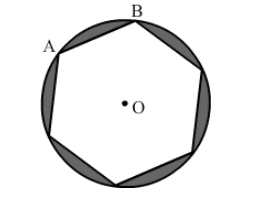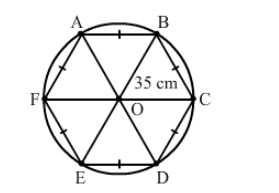# A round table cover has six equal designs as shown in the given figure.`
Question:

A round table cover has six equal designs as shown in the given figure. If the radius of the cover is 35 cm, then find the total area of the design.Solution:Join each vertex of the hexagon to the centre of the circle.

The hexagon is made up of six triangles.

Total area of the design = Area of the circle $-$ Area of six triangles

$=\left[\frac{22}{7} \times 35 \times 35\right]-\left[6 \times\left(\frac{\sqrt{3}}{4} \times 35 \times 35\right)\right]$

$=(35 \times 35)\left[\frac{22}{7}-\left(\frac{6 \times 1.732}{4}\right)\right]$

$=1225\left(\frac{22}{7}-2.598\right)$

$=1225 \times 0.542$

$=663.95 \mathrm{~cm}^{2}$